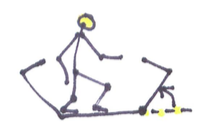Kinesthetics

Kinesthetic AnglesHenri Picciotto

At first, not all geometry students know what we're talking about when we talk about angles and their measures. This is probably why it is so difficult to teach some of them how to use a protractor: they don't know what they're measuring. Here are two kinesthetic activities that help a little, and follow-ups for Algebra 2 / Precalculus.

Arm Angles

Ask the students to stand up, and stick an arm straight out so that it is neither horizontal nor vertical. (This helps separate the idea of "straight" from the idea of "horizontal or vertical". Students often confuse those.) Then ask them to stick the other arm out, so that their arms make an acute angle, a right angle, an obtuse angle. It is surprising how difficult this is for some students.

A payoff down the line is that arm angles can be used to introduce the idea of the intercepted arc. The arc intercepted by this angle is the part of the circle I can see between my two arms. This may seem pointless, but a number of students have told me that this is what helped them see what was meant by the intercepted arc.

Walking polygons

This is based on turtle geometry as initially introduced by the Logo programming language, but it does not require a computer. A few "walking polygons" lessons are available in my Geometry Labs book. Here are two ideas:

• I tell students I will walk along the edge of an invisible parallelogram. Then I do the following, twice: two steps forward, a 135° turn to the left, one step forward, a 45° turn to the left. Of course it's not really possible for them to guess my turn angles, but it is entirely possible to figure out their sum, since after doing the two turns I'm facing in the opposite direction.
• Likewise, I tell them I'm going to walk a regular pentagon (or some other regular polygon -- preferably not a square.) At the end of the walk, I'm facing the way I faced at the beginning. This leads to a discussion of a formula for the size of the turn (or exterior) angle in a regular n-gon, and thus the size of the corresponding interior angle.

It is fun (and educational) to follow this up by programming a turtle in Logo or one of its descendants. (I use Snap, a Logo descendant by way of Scratch.)

The Unit Circle

I came across this one in The Mathematics Teacher(vol. 107, No. 6 February 2014, page 480), in an article by Maureen Macinnis, a teacher in Nova Scotia. It seems like a terrific activity, with a lasting impact.

She hands out pre-made cards, with the sine and cosine values for the sixteen "famous" angles (0°, 30°, 45°, and so on, all the way to 330°.) The sines are on orange cards, the cosines on green cards. Students are asked to pair up in sine-cosine pairs that correspond to one angle. For example, the student with an orange $\frac{{\sqrt 3 }}{2}$ needs to pair up with a green $\frac{1}{2}$ or $-\frac{1}{2}$. (That is already a good start!)

At this point, the lesson continues outside, where the teacher has pre-drawn a large chalk unit circle on the ground, with x and y axes. Student pairs find their position on the circle. This would be a good time to review who is at what angle.

But wait, there's more! The teacher has also drawn a pair of axes, with the y's from -1 to 1, and the x's marked off in degrees or radians. The next step is for the orange-card students to place themselves at the appropriate place on the graph, making a sine curve as the green-card students watch. And then they switch.